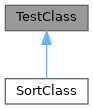ReactOS  0.4.14-dev-50-g13bb5e2
TestClass Class Reference

#include <TestClass.h>

Inheritance diagram for TestClass:[legend]

Public Member Functions

TestClass ()

TestClass (int value)

TestClass (const TestClass &rhs)

~TestClass ()

TestClassoperator= (const TestClass &rhs)

int value () const

TestClass operator! () const

bool operator== (const TestClass &rhs) const

bool operator< (const TestClass &rhs) const

Static Protected Member Functions

static unsigned int get_random (unsigned range=UINT_MAX)

Private Member Functions

void Init (int value)

intp

Detailed Description

Definition at line 32 of file TestClass.h.

◆ TestClass() [1/3]

 TestClass::TestClass ( )
inline

Definition at line 87 of file TestClass.h.

88 {
89  Init( int(get_random()) );
90 }
void Init(int value)
Definition: TestClass.h:77
static unsigned int get_random(unsigned range=UINT_MAX)
Definition: TestClass.h:154

Referenced by operator!().

◆ TestClass() [2/3]

 TestClass::TestClass ( int value )
inline

Definition at line 92 of file TestClass.h.

93 {
94  Init( value );
95 }
void Init(int value)
Definition: TestClass.h:77

◆ TestClass() [3/3]

 TestClass::TestClass ( const TestClass & rhs )
inline

Definition at line 97 of file TestClass.h.

98 {
99  Init( rhs.value() );
100 }
int value() const
Definition: TestClass.h:124
void Init(int value)
Definition: TestClass.h:77

◆ ~TestClass()

 TestClass::~TestClass ( )
inline

Definition at line 102 of file TestClass.h.

103 {
104 #if TESTCLASS_DEEP_DATA
105  delete p;
106 #else
108 #endif
109 }
void simulate_destructor()
Definition: nc_alloc.h:126
int * p
Definition: TestClass.h:60

◆ get_random()

 unsigned int TestClass::get_random ( unsigned range = UINT_MAX )
inlinestaticprotected

Definition at line 154 of file TestClass.h.

155 {
156  return random_number( range );
157 }
unsigned random_number(size_t range)
GLenum GLint * range
Definition: glext.h:7539

Referenced by TestClass().

◆ Init()

 void TestClass::Init ( int value )
inlineprivate

Definition at line 77 of file TestClass.h.

78 {
79 #if TESTCLASS_DEEP_DATA
80  p = new int( value );
81 #else
83  v = value;
84 #endif
85 }
int value() const
Definition: TestClass.h:124
void simulate_constructor()
Definition: nc_alloc.h:121
const GLdouble * v
Definition: gl.h:2040
GLfloat GLfloat p
Definition: glext.h:8902
unsigned int(__cdecl typeof(jpeg_read_scanlines))(struct jpeg_decompress_struct *
Definition: typeof.h:31

Referenced by TestClass().

◆ operator!()

 TestClass TestClass::operator! ( ) const
inline

Definition at line 133 of file TestClass.h.

134 {
135  return TestClass( value()+1 );
136 }
TestClass()
Definition: TestClass.h:87
int value() const
Definition: TestClass.h:124

◆ operator<()

 bool TestClass::operator< ( const TestClass & rhs ) const
inline

Definition at line 50 of file TestClass.h.

50  {
51  return value() < rhs.value();
52  }
int value() const
Definition: TestClass.h:124

◆ operator=()

 TestClass & TestClass::operator= ( const TestClass & rhs )
inline

Definition at line 111 of file TestClass.h.

112 {
113 #if TESTCLASS_DEEP_DATA
114  int *newP = new int( rhs.value() );
115  delete p;
116  p = newP;
117 #else
119  v = rhs.value();
120 #endif
121  return *this;
122 }
int value() const
Definition: TestClass.h:124
const GLdouble * v
Definition: gl.h:2040
void simulate_possible_failure()
Definition: nc_alloc.h:117
GLfloat GLfloat p
Definition: glext.h:8902
unsigned int(__cdecl typeof(jpeg_read_scanlines))(struct jpeg_decompress_struct *
Definition: typeof.h:31
int * p
Definition: TestClass.h:60

◆ operator==()

 bool TestClass::operator== ( const TestClass & rhs ) const
inline

Definition at line 45 of file TestClass.h.

46  {
47  return value() == rhs.value();
48  }
int value() const
Definition: TestClass.h:124

◆ value()

 int TestClass::value ( ) const
inline

Definition at line 124 of file TestClass.h.

125 {
126 #if TESTCLASS_DEEP_DATA
127  return *p;
128 #else
129  return v;
130 #endif
131 }
const GLdouble * v
Definition: gl.h:2040
int * p
Definition: TestClass.h:60

Referenced by Init(), operator !=(), operator!(), operator<(), operator=(), operator==(), and TestClass().

◆ p

 int* TestClass::p
private

Definition at line 60 of file TestClass.h.

Referenced by operator=(), value(), and ~TestClass().

The documentation for this class was generated from the following file: